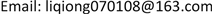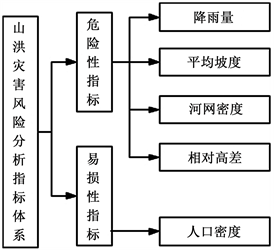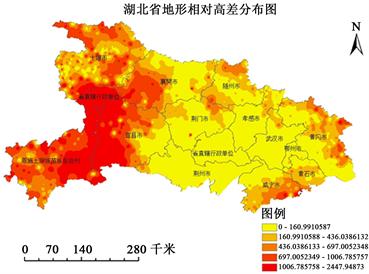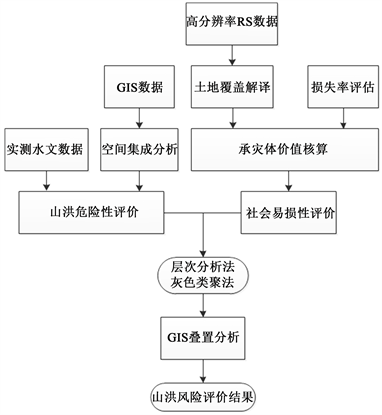﻿ 湖北省山洪灾害风险区的划分研究 Study on the Division of Mountain Flood Disaster Risk Zone in Hubei Province

Open Journal of Soil and Water Conservation
Vol. 06  No. 03 ( 2018 ), Article ID: 27951 , 11 pages
10.12677/OJSWC.2018.63004

Study on the Division of Mountain Flood Disaster Risk Zone in Hubei Province

Qiong Li1,2, Jianzhong Zhou2, Jian Zhou2

1Hubei Polytechnic University, Huangshi Hubei

2Institute of Hydropower and Digital Engineering, Huazhong University of Science and Technology, Wuhan HubeiReceived: Nov. 20th, 2018; accepted: Dec. 5th, 2018; published: Dec. 12th, 2018ABSTRACT

In this paper, the factors influencing the occurrence of mountain torrent disasters, such as rainfall, average slope, river network density and relative height difference, are selected and weighted to get the map of mountain torrent disaster risk degree in Hubei Province. The vulnerability analysis was carried out with population density and economic exposure as indicators. The hazard degree map and vulnerability map were superimposed to obtain the risk zoning map of mountain torrents in Hubei Province. On the basis of traditional research methods, remote sensing images combined with mountain torrent disaster census information are used to take full account of land use types, vegetation conditions, soil utilization and other information affecting flood discharge process, which makes the zoning results more reasonable and reliable. Comparing the data of historical torrential flood, it is found that the risk zoning of mountain flood has certain accuracy. Based on these results, the risk areas of mountain torrents can be planned, so as to mitigate the impact of mountain torrents, and provide disaster risk information for local residents.

Keywords:Risk Analysis, Grey Evaluation, Mountain Flood Disaster, Risk Zoning

1湖北理工学院，湖北 黄石

2华中科技大学，水电与数字化工程学院，湖北 武汉Copyright © 2018 by authors and Hans Publishers Inc.

This work is licensed under the Creative Commons Attribution International License (CC BY).

http://creativecommons.org/licenses/by/4.0/1. 引言

1.1. 风险分析的自然因素

1.2. 风险分析的社会经济要素

2. 风险分析指标体系建立Figure 1. Map of evaluation index system of mountain torrent disaster risk in Hubei Province

2.1. 山洪灾害危险性指标

2.1.1. 降雨因子Table 1. Basic characteristics of rainfall factors in Hubei Province

2.1.2. 地形因子Table 2. Basic characteristics of topographic factors

2.1.3. 平均坡度

2.1.4. 相对高差Figure 2. Distribution of relative height difference of topography in Hubei Province

2.1.5. 水系因子Table 3. Basic characteristics of water system factors

2.1.6. 河网密度

2.2. 山洪灾害易损性指标

2.2.1. 人口及经济暴露性Table 4. Basic characteristics of population and economic exposure

2.2.2. 人口密度

3. 山洪灾害风险评价方法及计算

3.1. 研究方法和技术路线

3.2. 湖北省山洪灾害风险评价流程Figure 3. Flow chart of flood risk zoning

4. 湖北省山洪灾害风险评价

4.1. 应用层次分析法确定因子权重Table 5. Judgment matrix of risk factors of mountain flood disastersTable 6. Weights of risk assessment factors for mountain torrents

4.2. 用灰色聚类评估方法确定风险等级

4.2.1. 建立梯形白化权函数进行综合评价确定评价对象灰类

${f}_{1}=\left\{\begin{array}{ll}1\hfill & x\in \left[0,80\right]\hfill \\ \frac{x-95}{-15}\hfill & x\in \left[80,95\right]\hfill \end{array}$ (1)

${f}_{2}=\left\{\begin{array}{ll}\frac{x-40}{40}\hfill & x\in \left[40,80\right]\hfill \\ 1\hfill & x\in \left[80,110\right]\hfill \\ \frac{x-125}{-15}\hfill & x\in \left[110,125\right]\hfill \end{array}$ (2)

${f}_{3}=\left\{\begin{array}{ll}\frac{x-95}{15}\hfill & x\in \left[95,110\right]\hfill \\ 1\hfill & x\in \left[110,140\right]\hfill \\ \frac{x-155}{-15}\hfill & x\in \left[140,155\right]\hfill \end{array}$ (3)

${f}_{4}=\left\{\begin{array}{ll}\frac{x-125}{15}\hfill & x\in \left[125,140\right]\hfill \\ 1\hfill & x\in \left[140,170\right]\hfill \\ \frac{x-185}{-15}\hfill & x\in \left[170,185\right]\hfill \end{array}$ (4)

${f}_{5}=\left\{\begin{array}{ll}1\hfill & x\in \left[170,\infty \right]\hfill \\ \frac{x-155}{15}\hfill & x\in \left[155,170\right]\hfill \end{array}$ (5)

4.2.2. 计算灰色评价权矩阵

${x}_{i}^{\left(s\right)}=\underset{e=1}{\overset{15}{\sum }}{x}_{ie}^{\left(s\right)}$ (6)

${r}_{ie}^{\left(s\right)}=\frac{{x}_{ie}^{\left(s\right)}}{{x}_{i}^{\left(s\right)}}$ (7)

${r}_{i}^{s}=\left({r}_{i1}^{\left(s\right)},{r}_{i2}^{\left(s\right)},\cdots ,{r}_{i15}^{\left(s\right)}\right)$ (8)

${R}^{\left(s\right)}=\left(\begin{array}{cccc}{r}_{1,1}^{\left(s\right)}& {r}_{1,2}^{\left(s\right)}& \cdots & {r}_{1,5}^{\left(s\right)}\\ {r}_{2,1}^{\left(s\right)}& {r}_{2,2}^{\left(s\right)}& \cdots & {r}_{2,5}^{\left(s\right)}\\ ⋮& ⋮& \ddots & ⋮\\ {r}_{15,1}^{\left(s\right)}& {r}_{15,2}^{\left(s\right)}& \cdots & {r}_{15,5}^{\left(s\right)}\end{array}\right)$ (9)

4.3. GIS软件的应用

5. 山洪灾害风险区划与成果检验

$R=0.8H+0.2V$Table 8. Risk classification of mountain flood disasters in Hubei ProvinceTable 9. The proportion of township area affected by mountain torrents

6. 结论与展望

Study on the Division of Mountain Flood Disaster Risk Zone in Hubei Province[J]. 水土保持, 2018, 06(03): 19-29. https://doi.org/10.12677/OJSWC.2018.63004

1. 1. 仇蕾, 王慧敏, 马树建. 极端洪水灾害损失评估方法及应用[J]. 水科学进展, 2009, 20(6): 869-875.

2. 2. 魏一鸣, 金菊良, 杨存建, 等. 洪水灾害风险管理理论[M]. 北京: 科学出版社, 2002: 11-18.

3. 3. Zou, Q., Zhou, J.Z., Zhou, C., et al. (2013) Comprehensive Flood Risk Assessment Based on Set Pair Analysis-Variable Fuzzy Sets Model and Fuzzy AHP. Stochastic Environmental Research and Risk Assessment, 27, 525-546.

4. 4. Li, Q., Zhou, J.Z., Liu, D.H., et al. (2012) Disaster Risk Assessment Based on Variable Fuzzy Sets and Improved Information Diffusion Method. Human and Ecological Risk Assessment, 19, 857-872.

5. 5. 汪志红, 王斌会. 投影寻踪技术在突发事件风险分类评级中的应用-以广东省雷电灾害风险评价为例[J]. 灾害学, 2011, 26(3): 78-82.

6. 6. 刘雨华. 基于梯形模糊数的指标权重确定方法的应用研究[J]. 南京信息工程大学学报: 自然科学版, 2009, 1(4): 369-372.

7. 7. 赵昱, 刘行, 徐佳欣, 等. 基于层次分析法—灰色聚类的PFI模式下公租房建设的风险评价方法[J]. 工程管理学报, 2013, 27(1): 69-72.

8. 8. 丁文峰, 杜俊, 陈小平, 等. 四川省山洪灾害风险评估与区划[J]. 长江科学院院报, 2015, 32(12): 41-45.